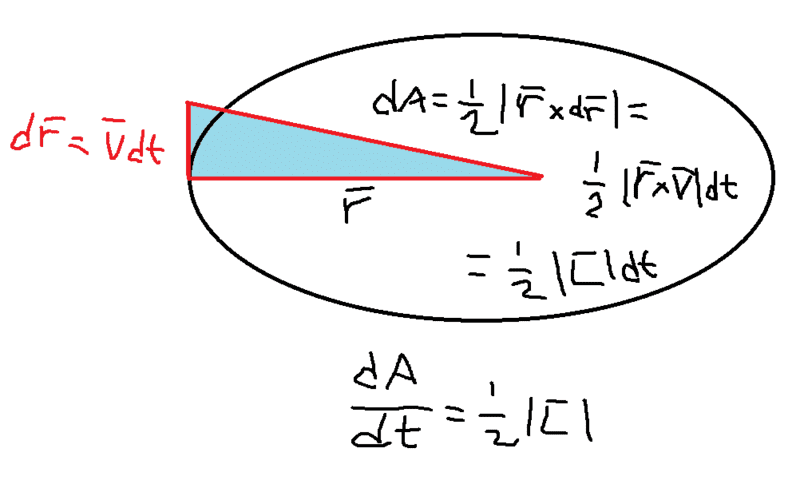# Kepler's second law derivation from angular momentum conservation

• I
• mr_sparxx

#### mr_sparxx

TL;DR Summary
Many texts state that Kepler's second law can be derived from conservation of angular momentum, however all demonstrations I have found make an assumption that is not clear for me
Many texts state that in an elliptic orbit you can find angular momentum magnitude as

$$L = r m v = m r^2 \frac {d \theta} {dt}$$

I wonder if
$$v = r \frac {d \theta} {dt}$$

is valid at every point. I understand this approximation in a circumference or radius r but what about an arc of ellipse?

Thank you.

•malawi_glenn

I usually explain it like this (dr vector is exaggerated for the sake of the picture)Where I used that the area of the entire parallellogram formed by the r and dr vectors is equal to the magnitude of their cross product. Here we have half a parallellogram, hence the facor 1/2

Here it is with LaTex:
##\mathrm{d}\vec r = \vec v \mathrm{d}t##
## \mathrm{d} A = \frac{1}{2}| \vec r \times \mathrm{d}\vec r| = \frac{1}{2}| \vec r \times \vec v|\mathrm{d}t =\frac{1}{2}| \vec L |\mathrm{d}t ##

If L is conserved then ## \dfrac{\mathrm{d}A}{\mathrm{d}t}## is constant and thus by integrating it we get the area swept out during a certain time interval is constant along the path

Last edited by a moderator:
••Delta2, mr_sparxx and vanhees71
Just to point out that this is not particular to the Kepler potential. It is true for any central potential as angular momentum ##L = mr^2\dot\theta## is conserved due to the rotational symmetry.

•mr_sparxx, vanhees71 and malawi_glenn
It was also important for the Bohr quantum model of the hydrogen atom. Since the potential is spherical symmetric, angular momentm is conserved. Idea was to hypothesize that electrons bound to proton only exhibit certain angular momenta, namely an integer multiple of Plancks constant / 2 (and was it a factor of π as well somewhere?)

The true spirit of the Bohr-Sommerfeld model is that for (quasi-)periodic motion the action is quantized in multiples of ##h=2 \pi \hbar##. This implies a quantization of angular-momentum components as integer multiples of ##\hbar## since angular momentum is the canonical conjugate to angle variables.

•malawi_glenn
I usually explain it like this (dr vector is exaggerated for the sake of the picture)
View attachment 304176
Where I used that the area of the entire parallellogram formed by the r and dr vectors is equal to the magnitude of their cross product. Here we have half a parallellogram, hence the facor 1/2

Here it is with LaTex:
##\mathrm{d}\vec r = \vec v \mathrm{d}t##
## \mathrm{d} A = \frac{1}{2}| \vec r \times \mathrm{d}\vec r| = \frac{1}{2}| \vec r \times \vec v|\mathrm{d}t =\frac{1}{2}| \vec L |\mathrm{d}t ##

If L is conserved then ## \dfrac{\mathrm{d}A}{\mathrm{d}t}## is constant and thus by integrating it we get the area swept out during a certain time interval is constant along the path

I guess you mean ( mass is missing)
## \mathrm{d} A = \frac{1}{2}| \vec r \times \mathrm{d}\vec r| = \frac{1}{2}| \vec r \times \vec v|\mathrm{d}t =\frac{1}{2m}| \vec L |\mathrm{d}t ##

Nice way of explaining it. Just what I was looking for. Thank you.

•Delta2, malawi_glenn and vanhees71
I guess you mean ( mass is missing)
## \mathrm{d} A = \frac{1}{2}| \vec r \times \mathrm{d}\vec r| = \frac{1}{2}| \vec r \times \vec v|\mathrm{d}t =\frac{1}{2m}| \vec L |\mathrm{d}t ##

Nice way of explaining it. Just what I was looking for. Thank you.
Yes I forgot mass.

•Delta2 and vanhees71